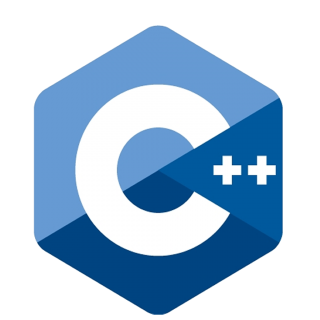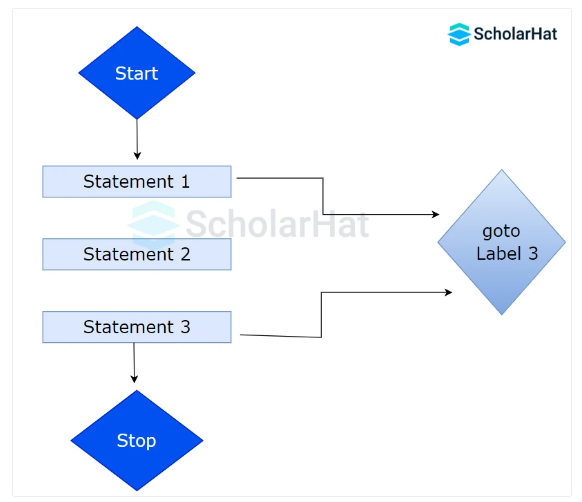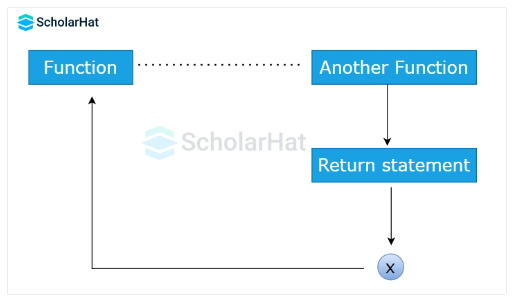# Goto and Return Statements in C++

24 Nov 2023
Beginner
95 ViewsLearn via Video Course & by Doing Hands-on Labs

## Goto and Return Statements in C++: An Overview

In the previous C++ tutorial on jump statements, we saw the break and continue statements in C++ in complete detail. It's now time to move on to the other two important jump statements, `goto` and `return` statements in C++. If you want to become a certified C++ programmer, you can also consider our C++ Certification Course.

## Goto Statement in C++

It is a control flow mechanism that enables programmers to move the execution of a program to a particular `labeled` statement inside the code. The `goto` statement in C++ allows one to skip over the customary sequential flow of the code and go to a specific location within it. The best practice is to avoid the requirement for `goto` statements whenever possible by using structured programming elements like loops and conditionals.#### Syntax

``````goto label;
// ...
label:
// Statement(s) to execute
``````

#### Example to demonstrate goto statement in C++

``````#include <iostream>
using namespace std;
int main() {
int choice;
cout << "Select an option:" << endl;
cout << "1. Print 'Hello'" << endl;
cout << "2. Print 'World'" << endl;
cout << "3. Exit" << endl;
cin >> choice;

switch (choice) {
case 1:
cout << "Hello" << endl;
break;
case 2:
cout << "World" << endl;
break;
case 3:
cout << "Exiting..." << endl;
default:
cout << "Invalid choice" << endl;
break;
}

// This is where the 'end' label is defined
end:
cout << "Program ends here." << endl;
return 0;
}
``````
• This C++ program gives the user a menu to select an option.
• Depending on what they select, the program either prints "Hello," "World," or ends.
• When the user chooses the `exit` option `3`, the `goto` statement is used to leap to the `end` label, enabling the program to end and output "Programme ends here."

#### Output

``````Select an option:
1. Print 'Hello'
2. Print 'World'
3. Exit
``````

• If the user enters 1st choice

#### Output

``````Hello
Program ends here.
``````
• If the user enters 2nd choice

#### Output

``````World
Program ends here.
``````
• If the user enters 3rd choice

#### Output

``````Exiting...
Program ends here.
``````

## Return Statement in C++

When a function is finished running, the `return` statement in C++ is used to give the caller a value. It is often used to return a result to the caller code.#### Syntax

``````return expression;
``````

#### Example to demonstrate return statement in C++

`````` #include <iostream>
using namespace std;
int multiply(int a, int b) {
return a * b;
}

int main() {
int result = multiply(5, 7);
cout << "The product is: " << result << endl;
return 0;
} ``````
• Here, the `main()` function calls the `multiply()` function to multiply the values 5 & 7.
• The `multiply()` function multiplies the values and returns the product to the `main()` function.

#### Output

``````The product is: 35
``````

Discover more about `functions` and use of the `return` statement in the section, Functions in C++, Call by Value and Call by Reference in C++.

##### Summary
With this, we have now completed all four jump statements in C++. You will now see the use of these statements in our further tutorials on C++. As we have already seen these statements in much detail, you won't have a problem understanding their usage. For a more in-depth understanding, consider our C++ Certification Course.
Share Article
Similar Articles